# NCERT Exemplar Class 12 Physics Solutions for Chapter 2 - Electrostatic Potential And Capacitance

NCERT Exemplar Solutions for Class 12 Physics Chapter 2 Electrostatic Potential and Capacitance give accurate information and knowledge in easy-to-understand language. By solving the NCERT Physics exemplar problems available at BYJU’S, you will be able to grasp the topics covered in Chapter 2, Electrostatic Potential and Capacitance, thoroughly.

NCERT Class 12 Physics Exemplar Chapter 2 is an important study resource for the students as it consists of questions from the NCERT Exemplar Class 12 Physics book. NCERT Class 12 Physics Exemplar for Chapter 2 Electrostatic Potential and Capacitance PDF has extra questions prepared by our subject experts in different forms like fill in the blanks, match the following, short and long answer questions, true or false questions, etc.

## Download the PDF of NCERT Exemplar Solutions for Class 12 Physics Chapter 2 Electrostatic Potential and Capacitance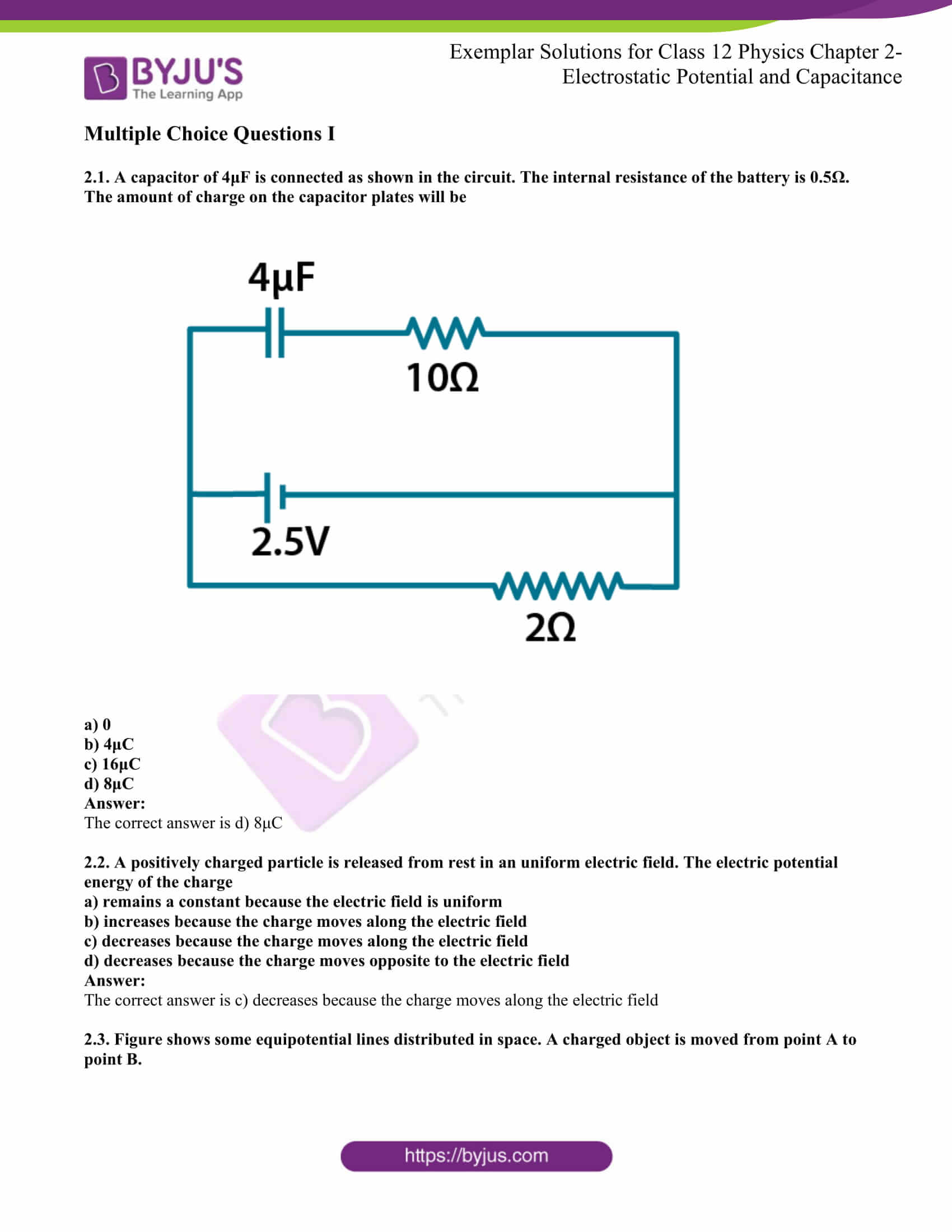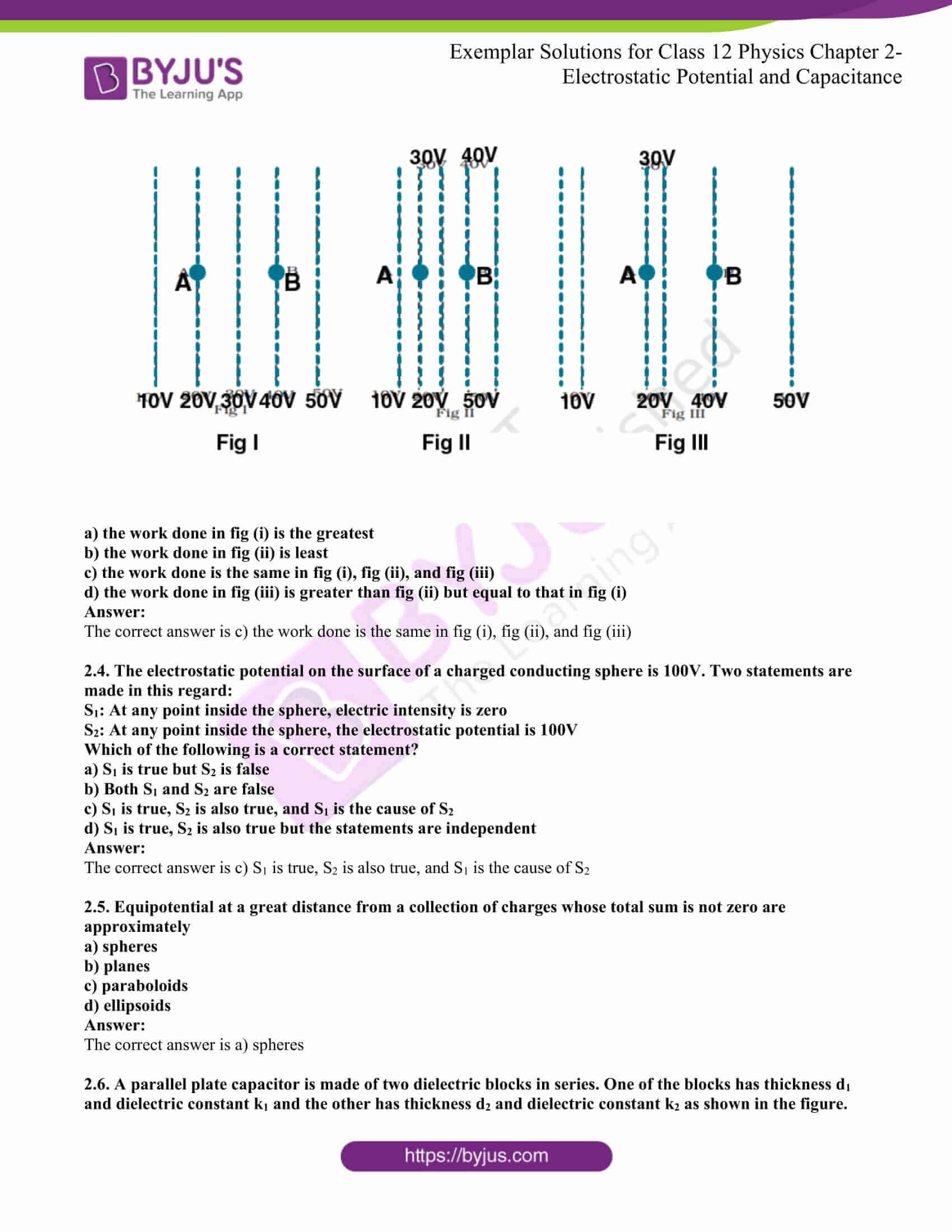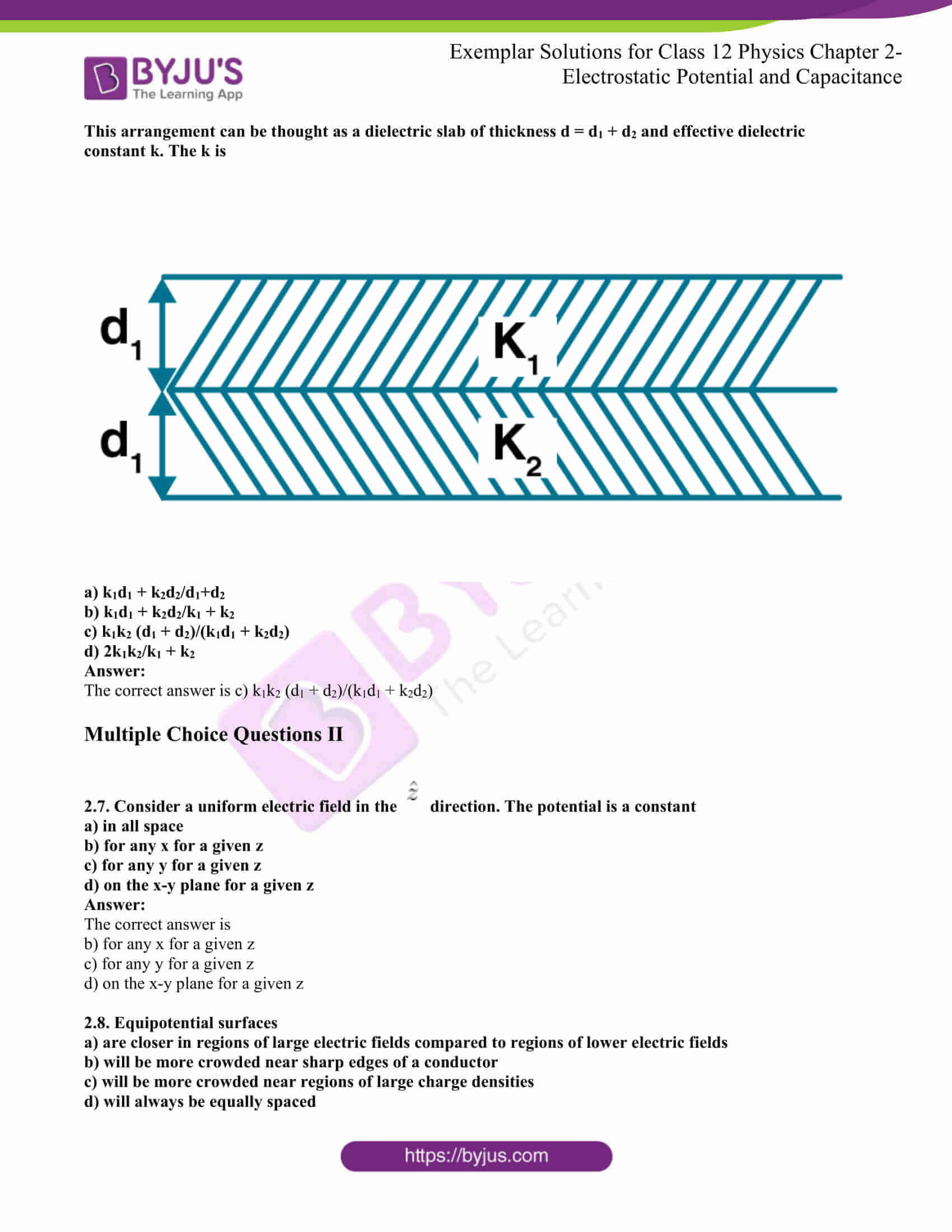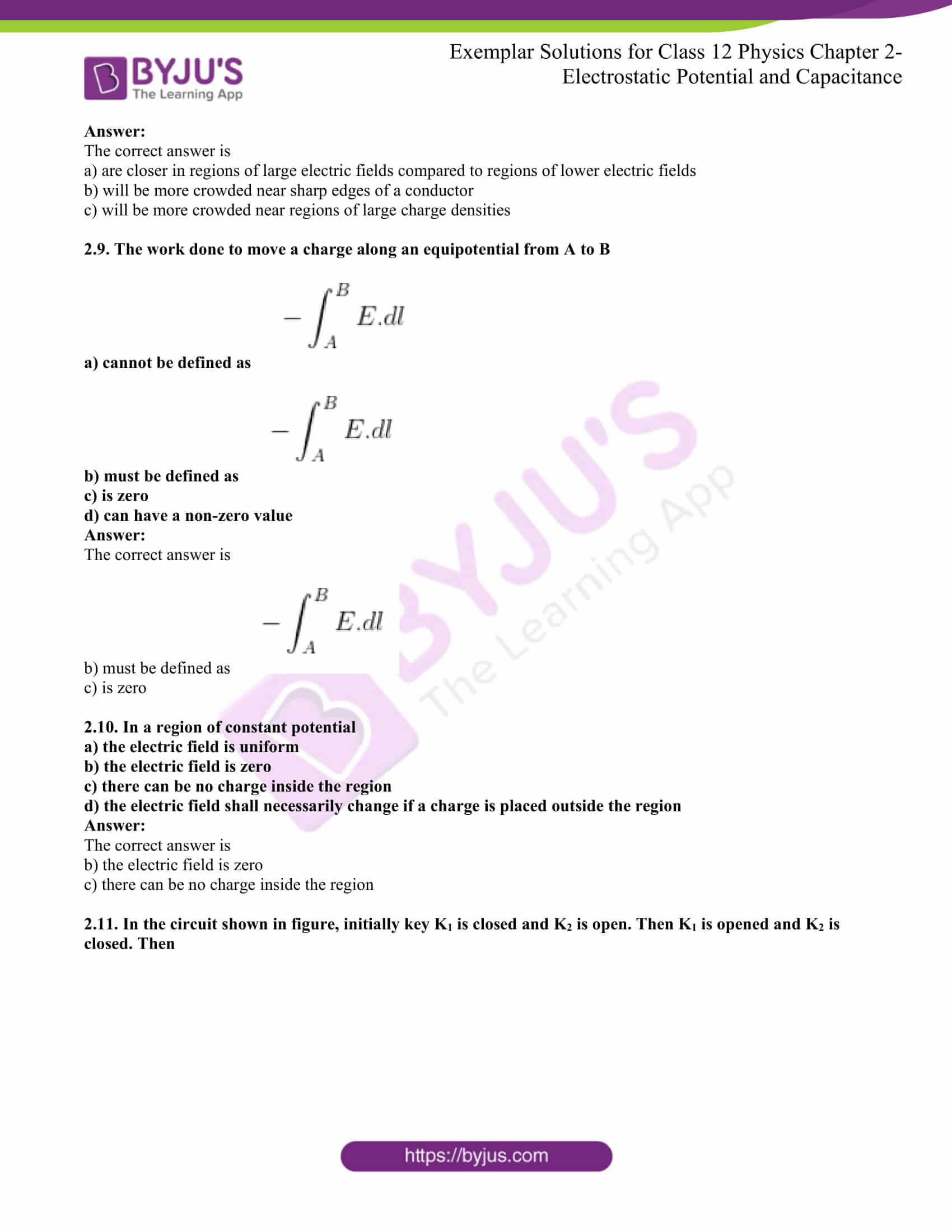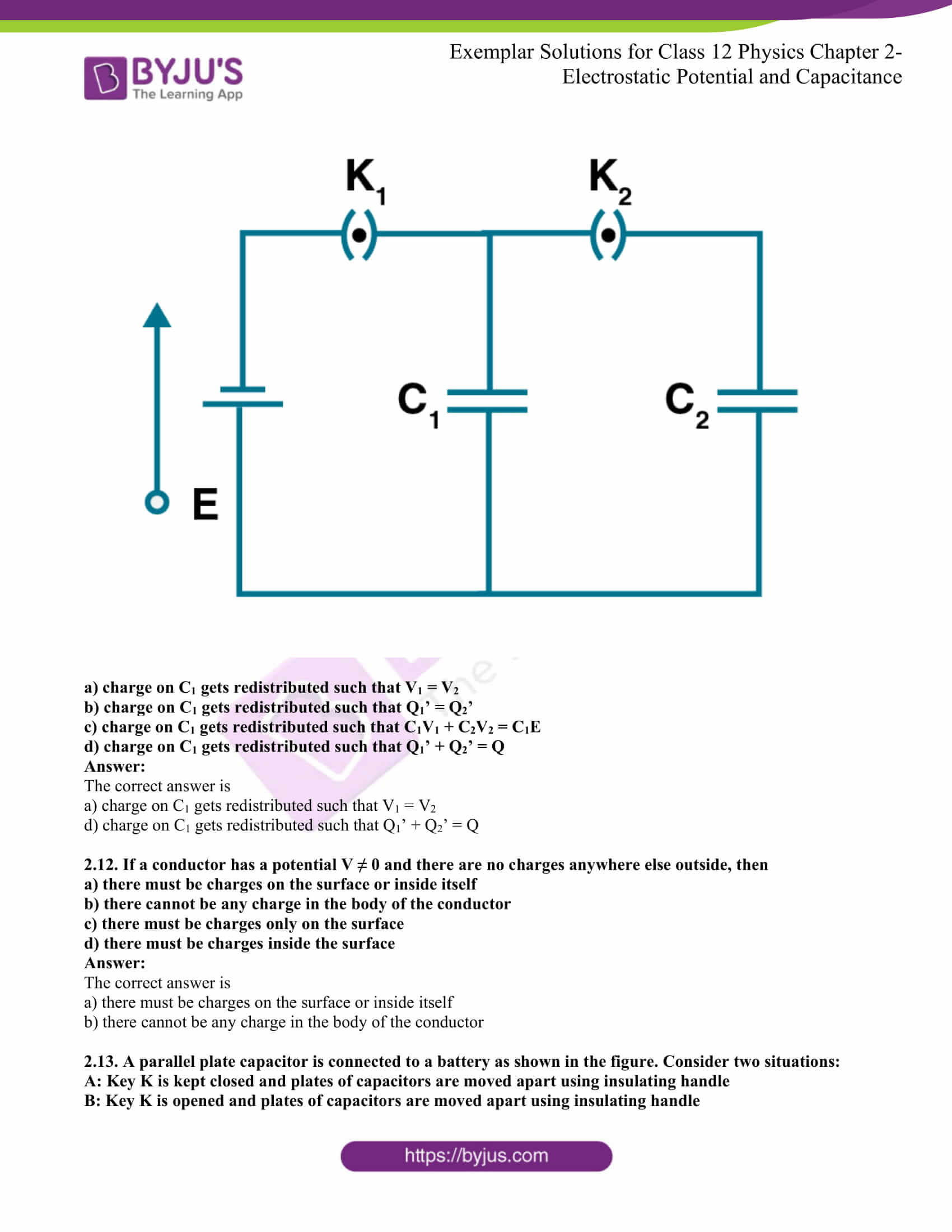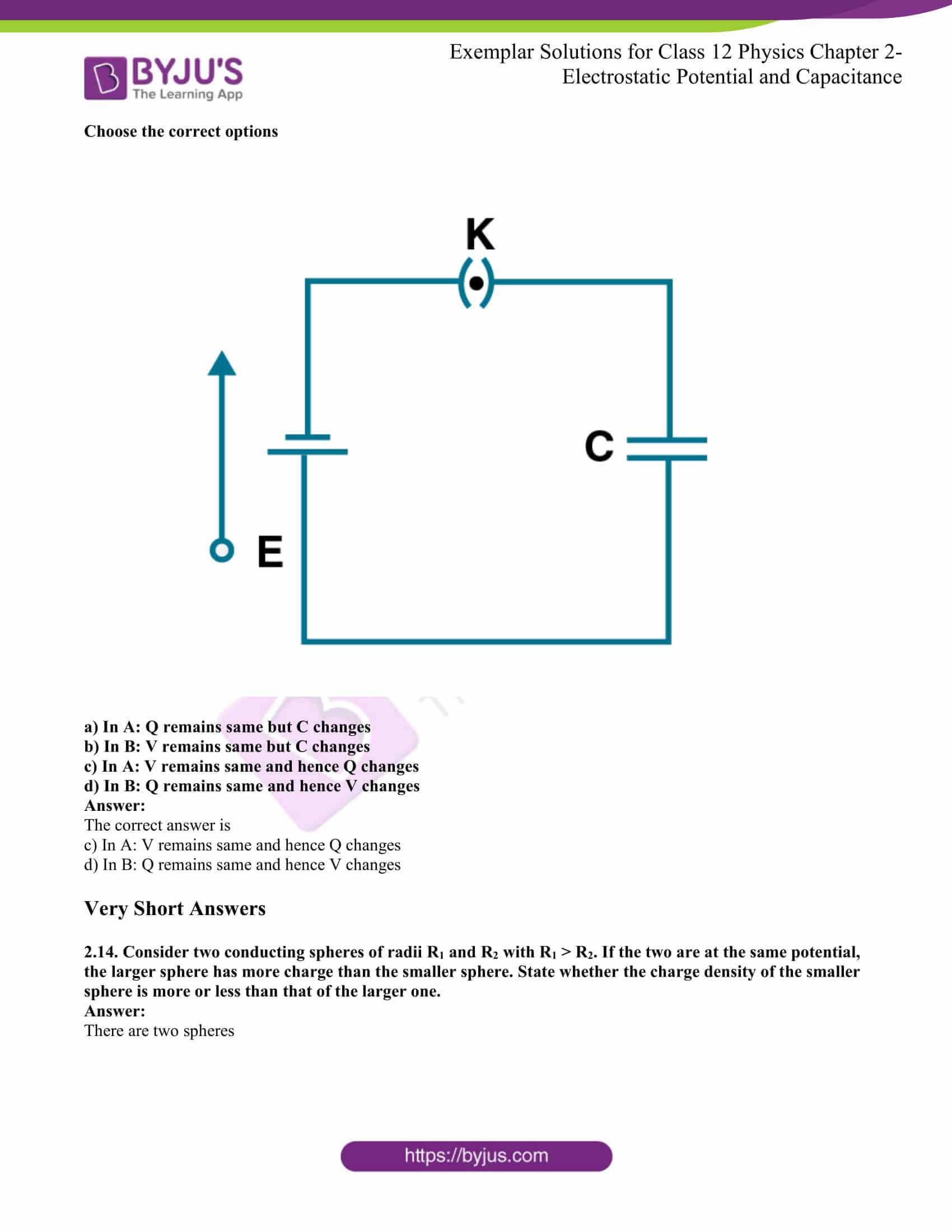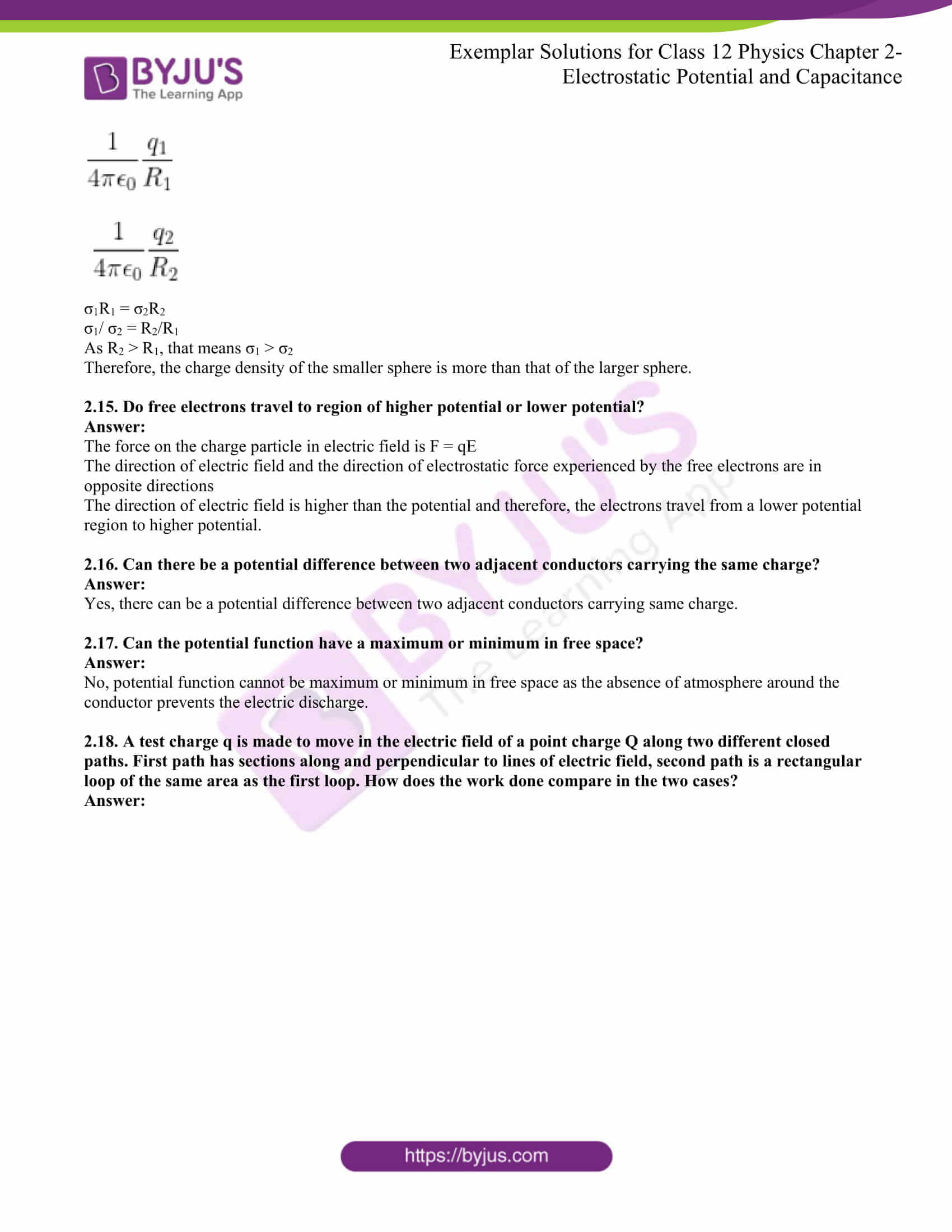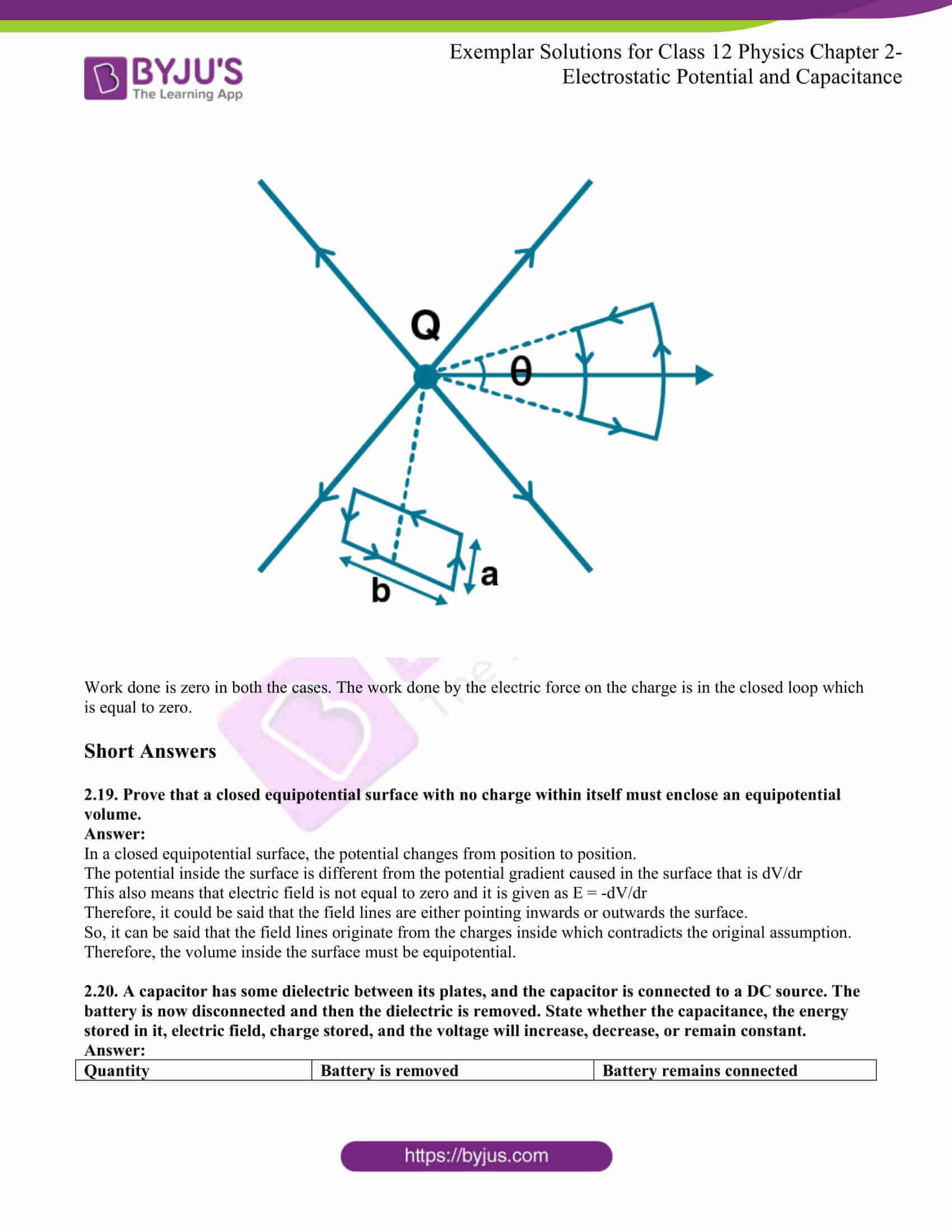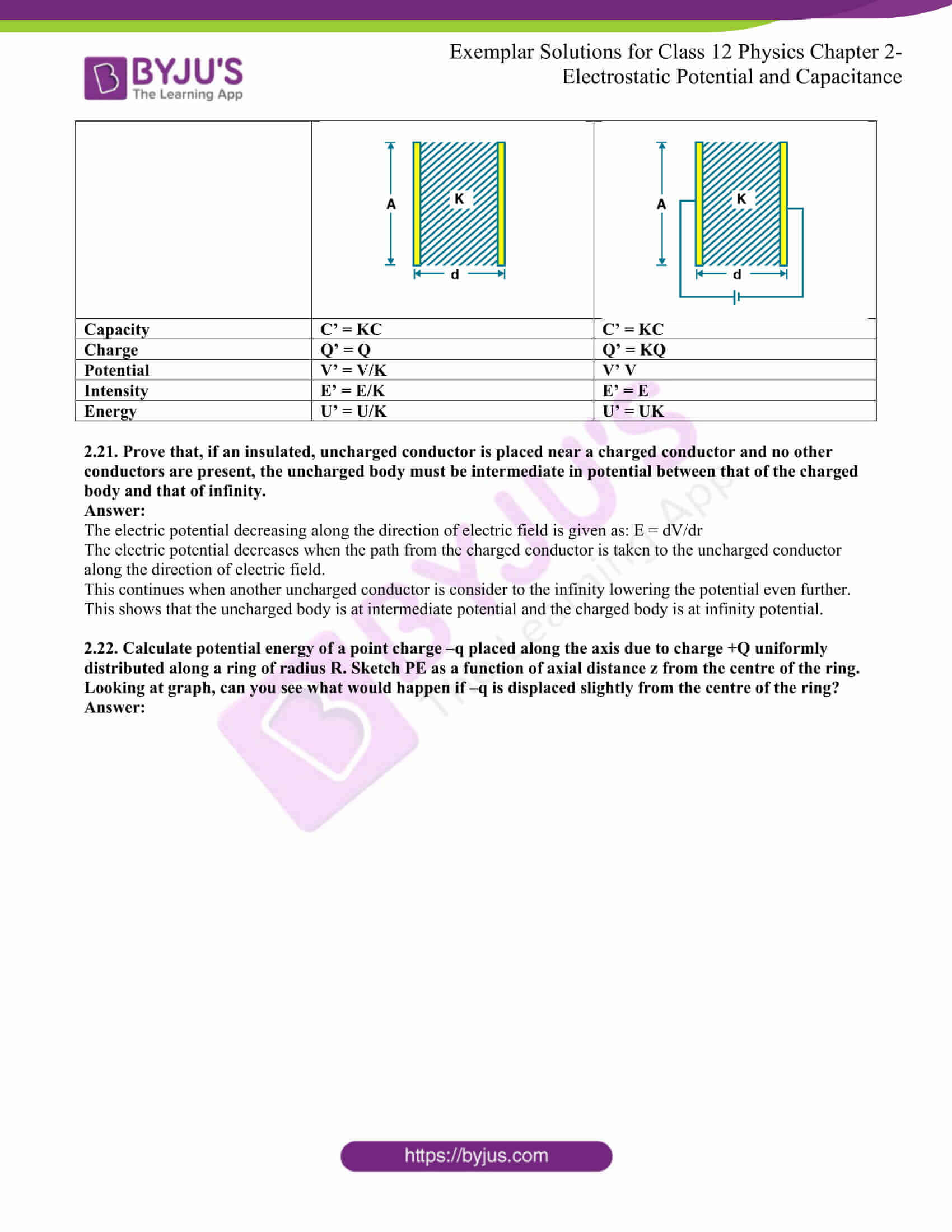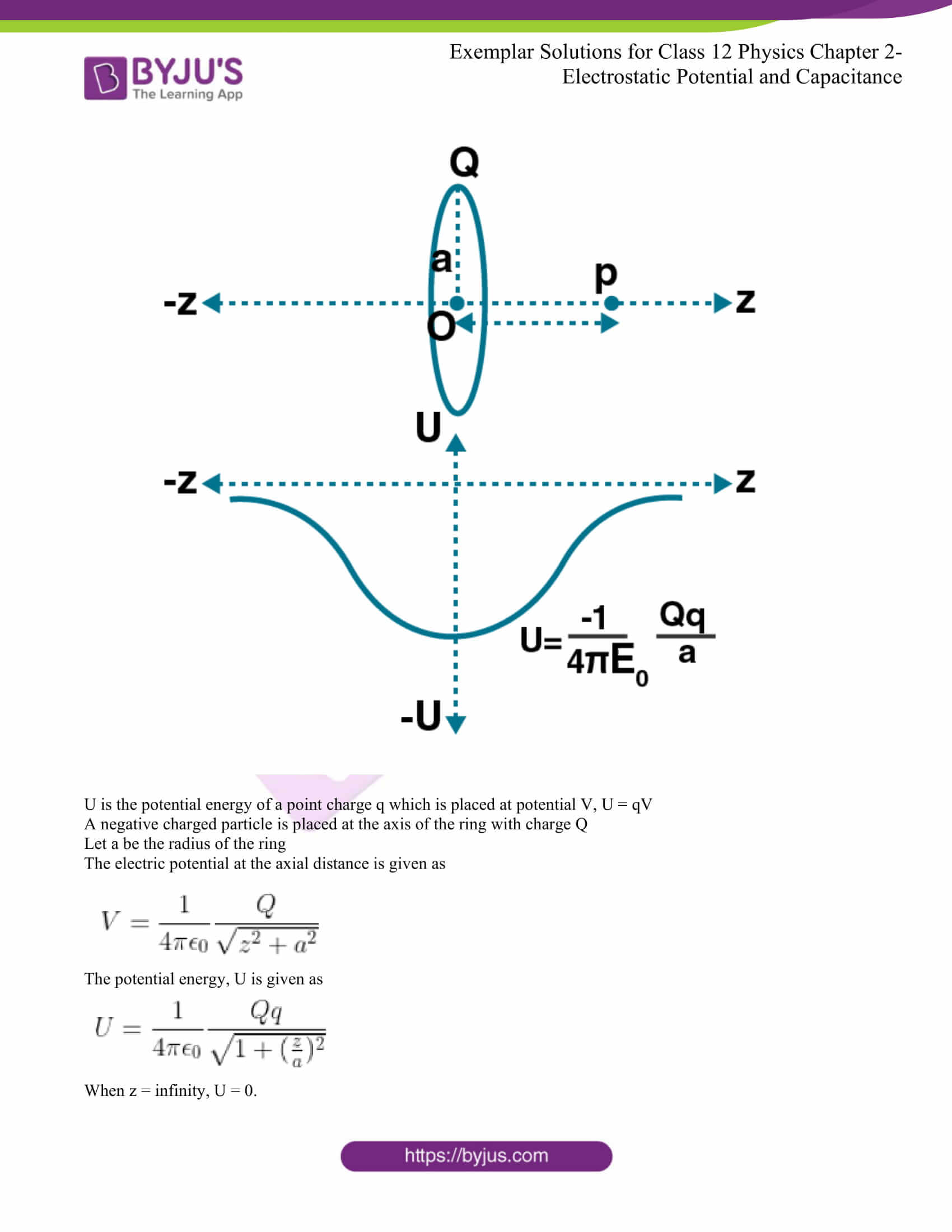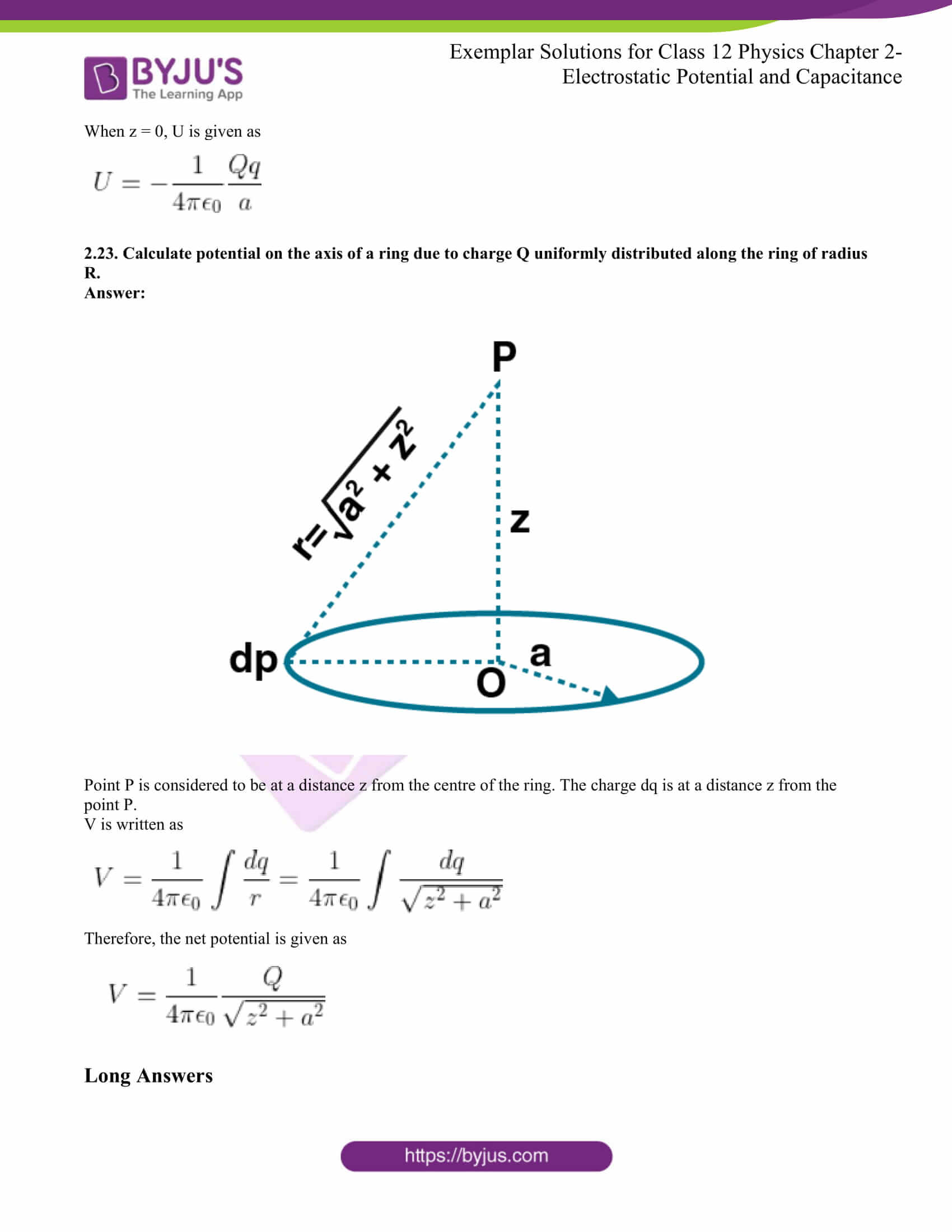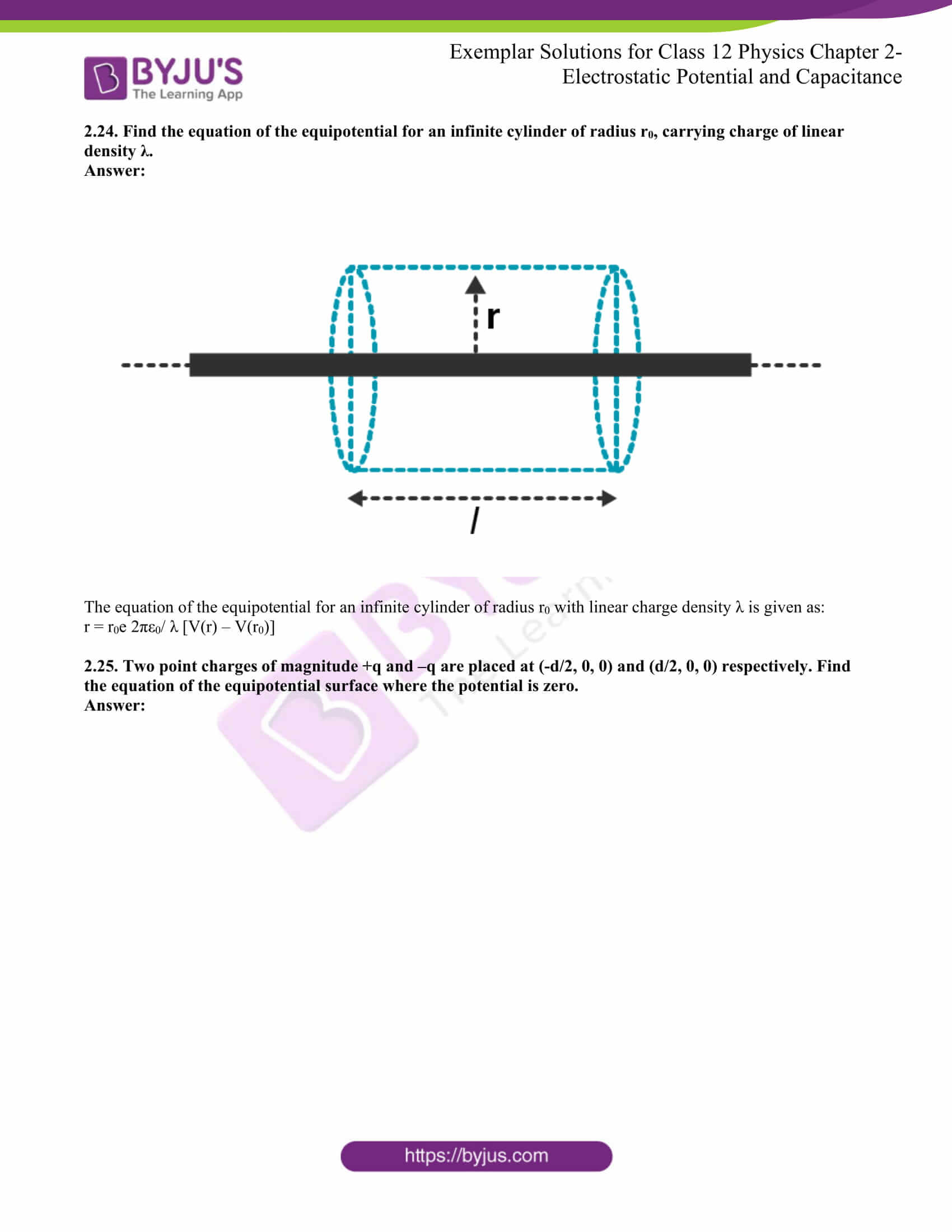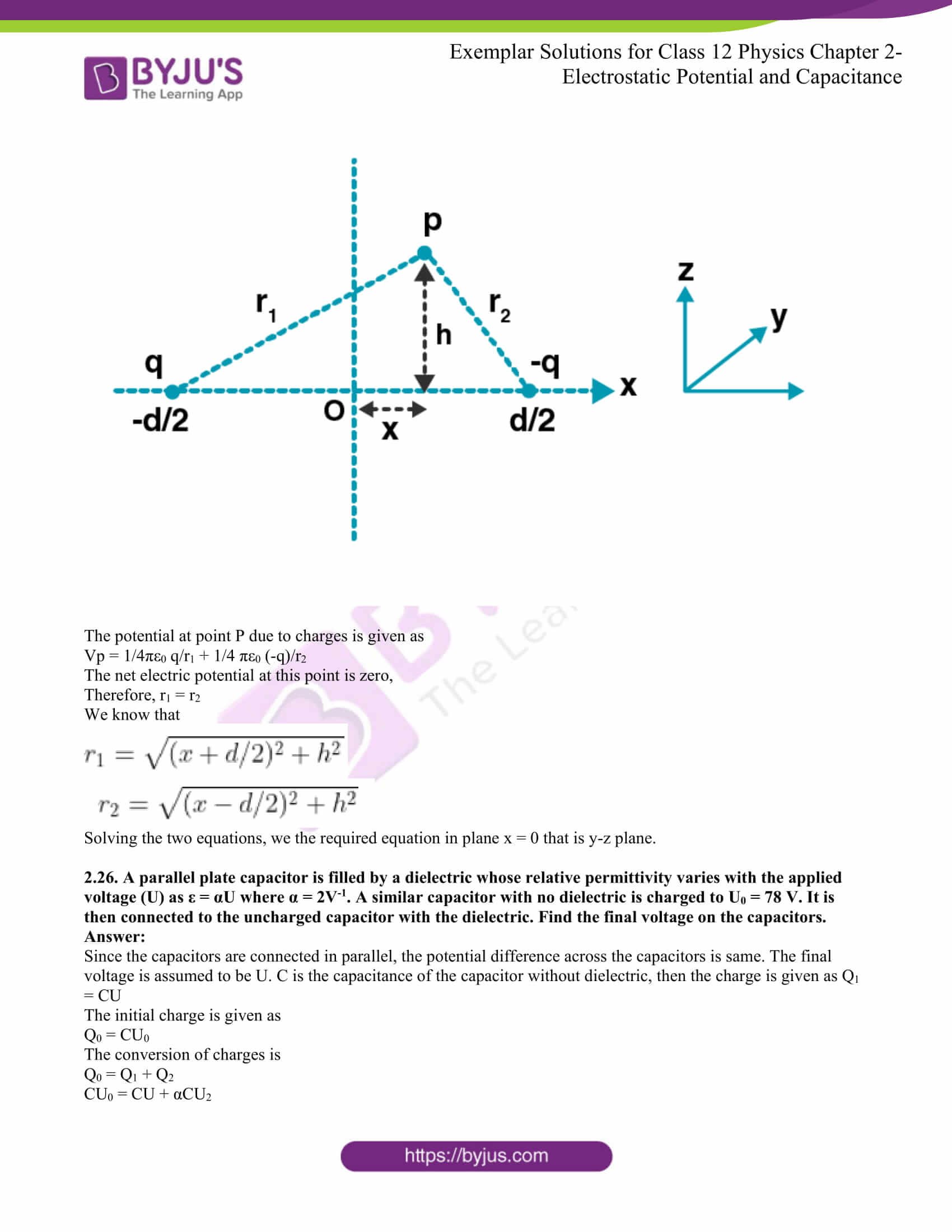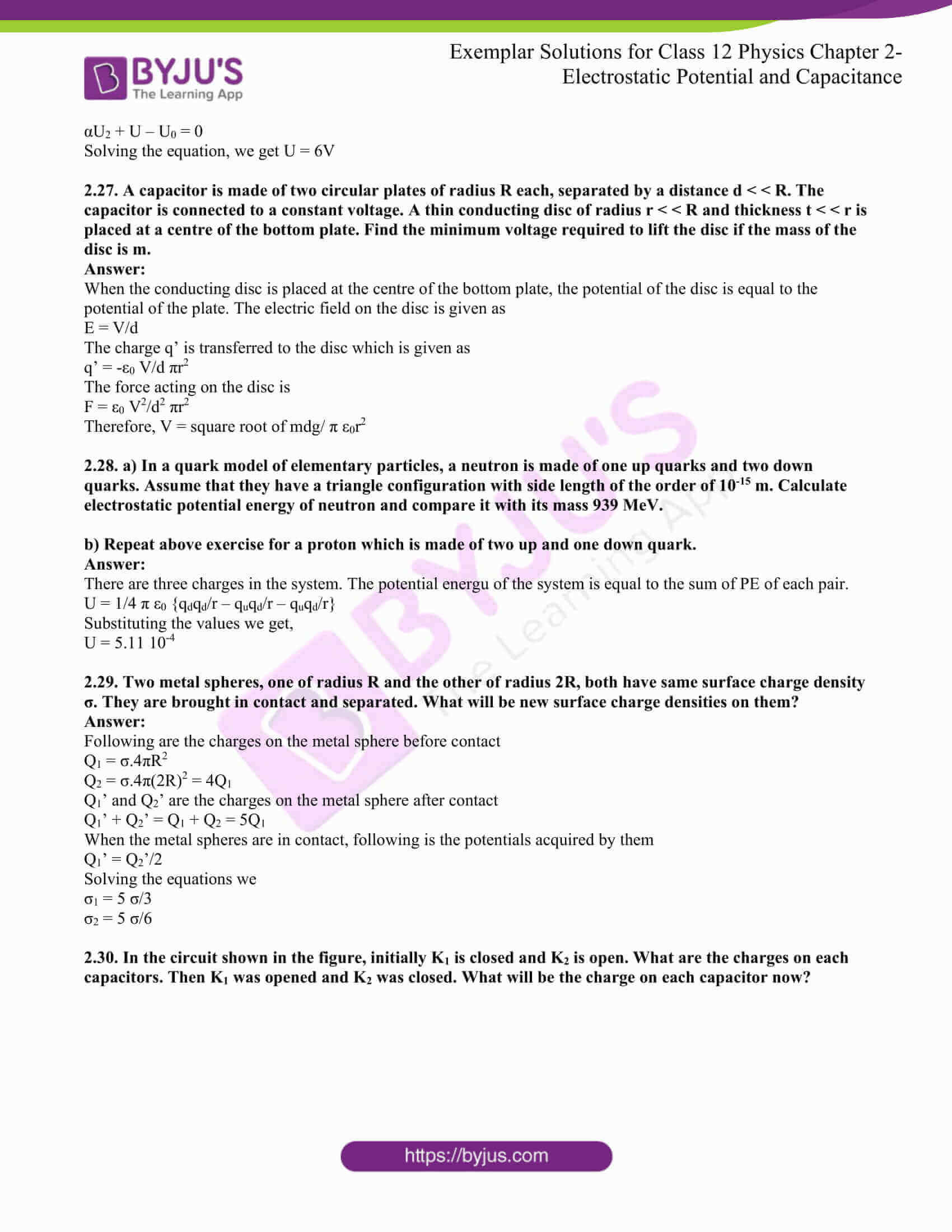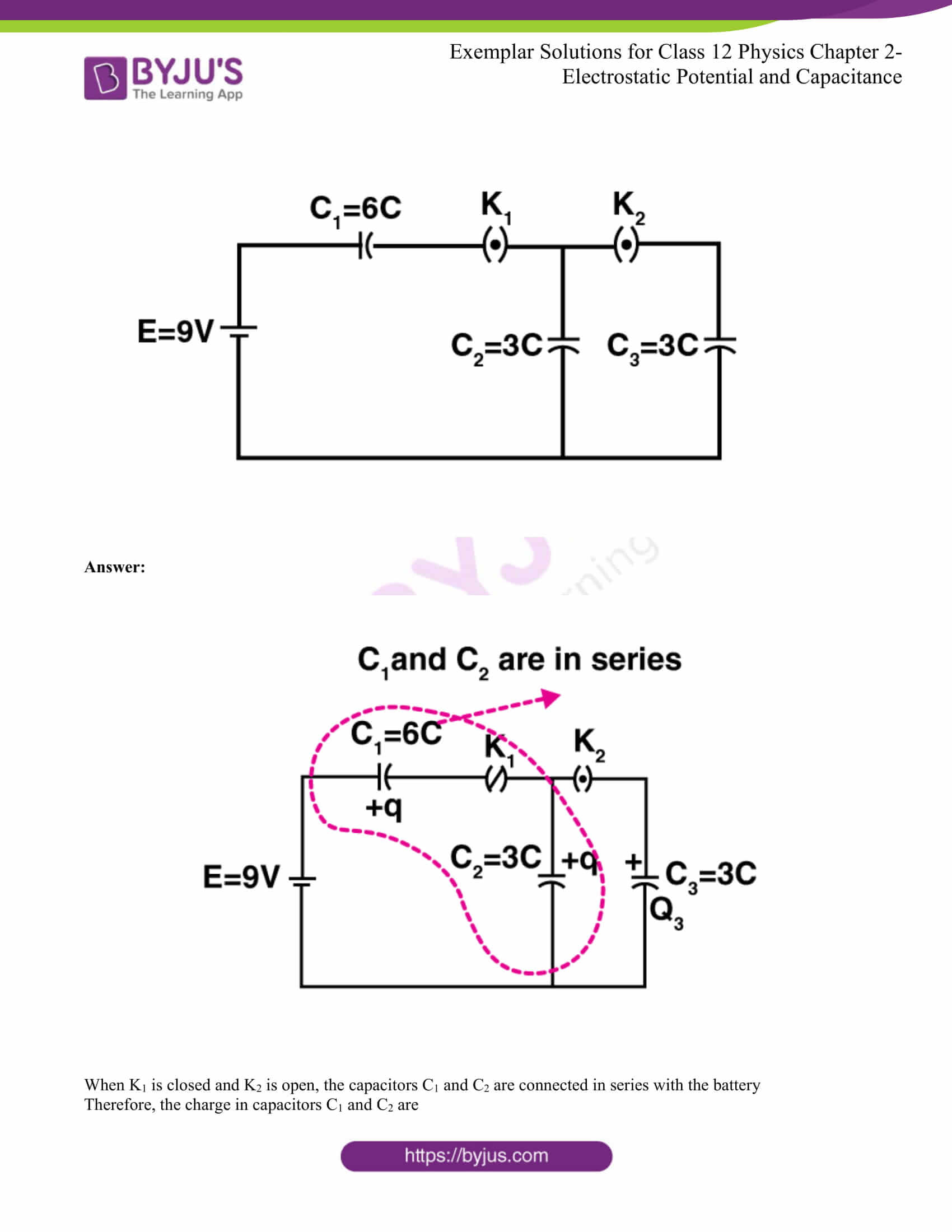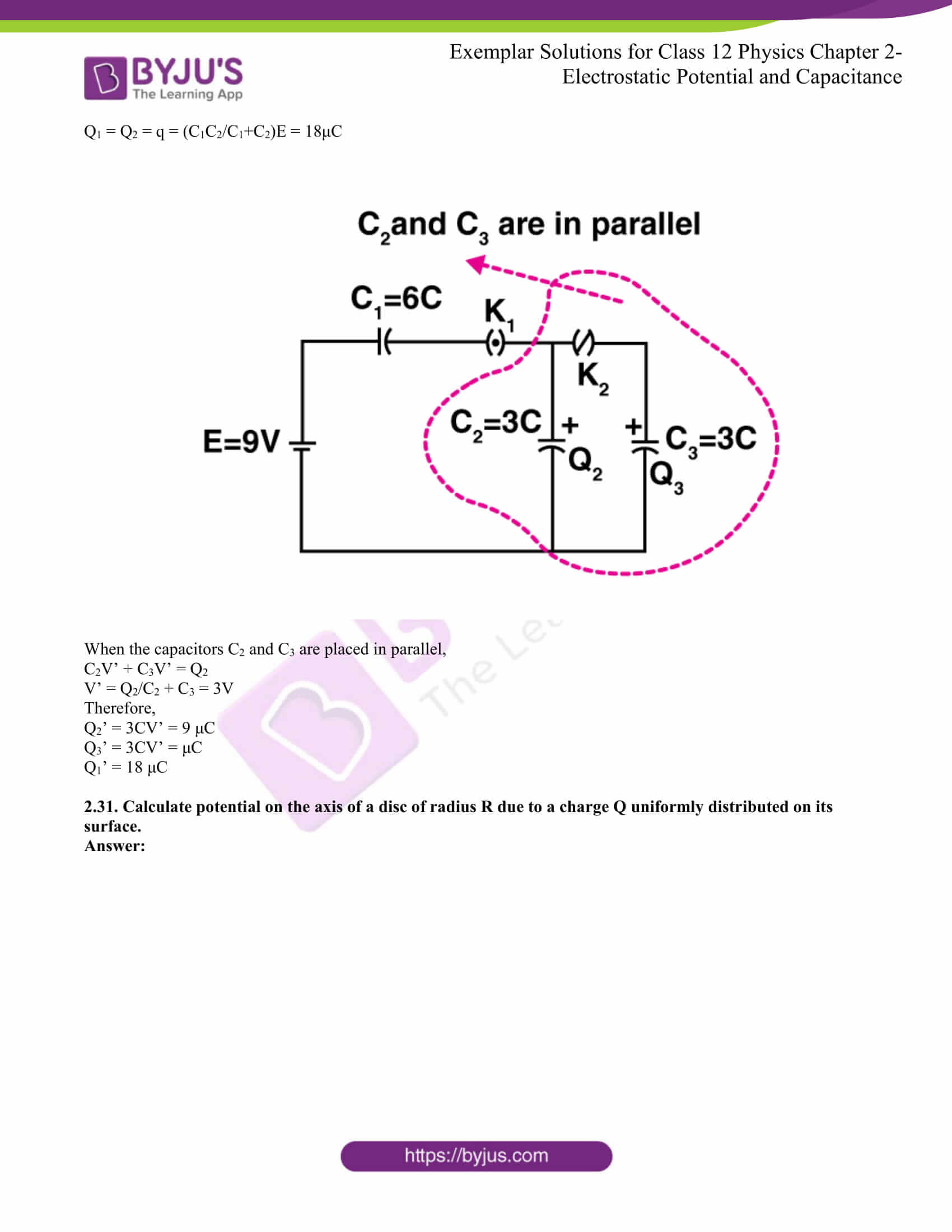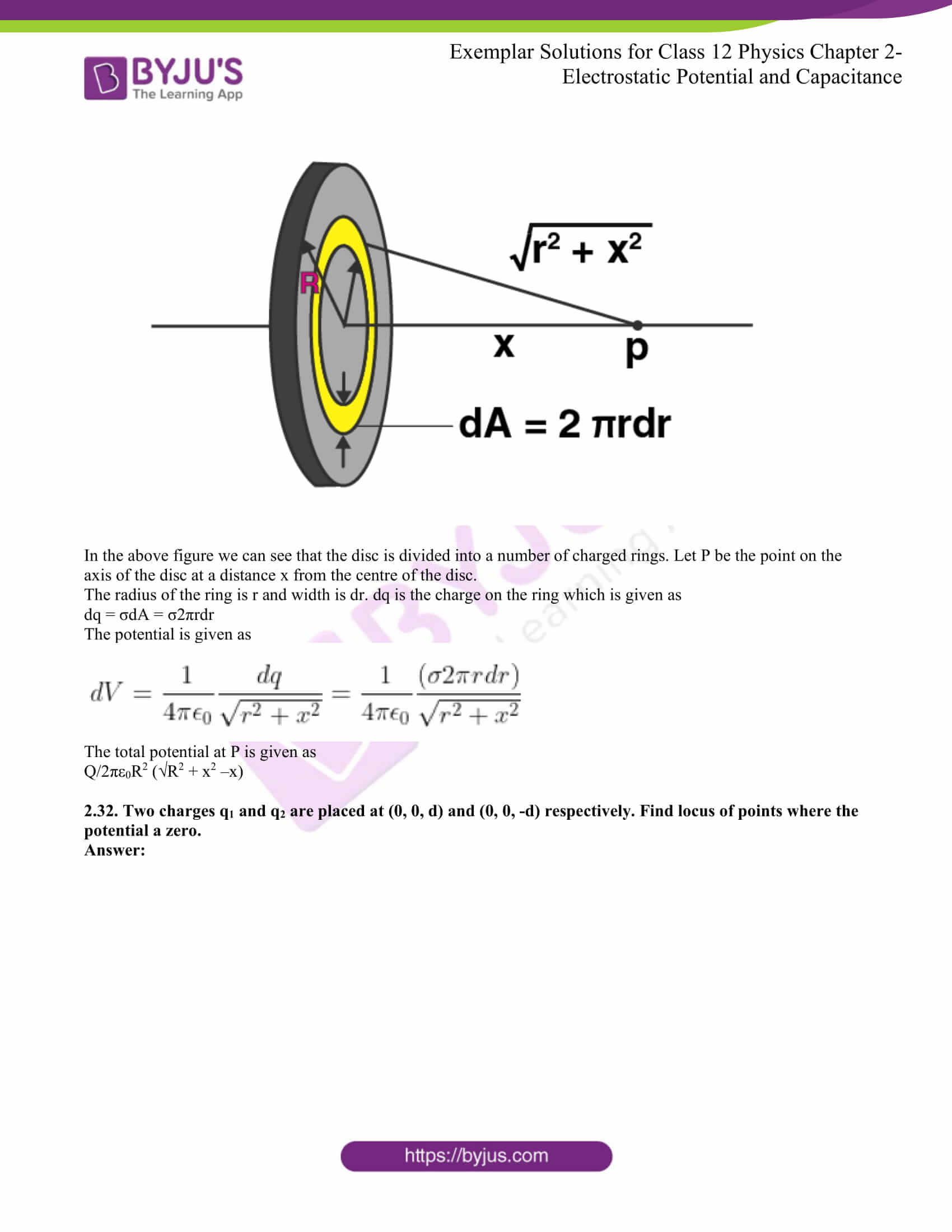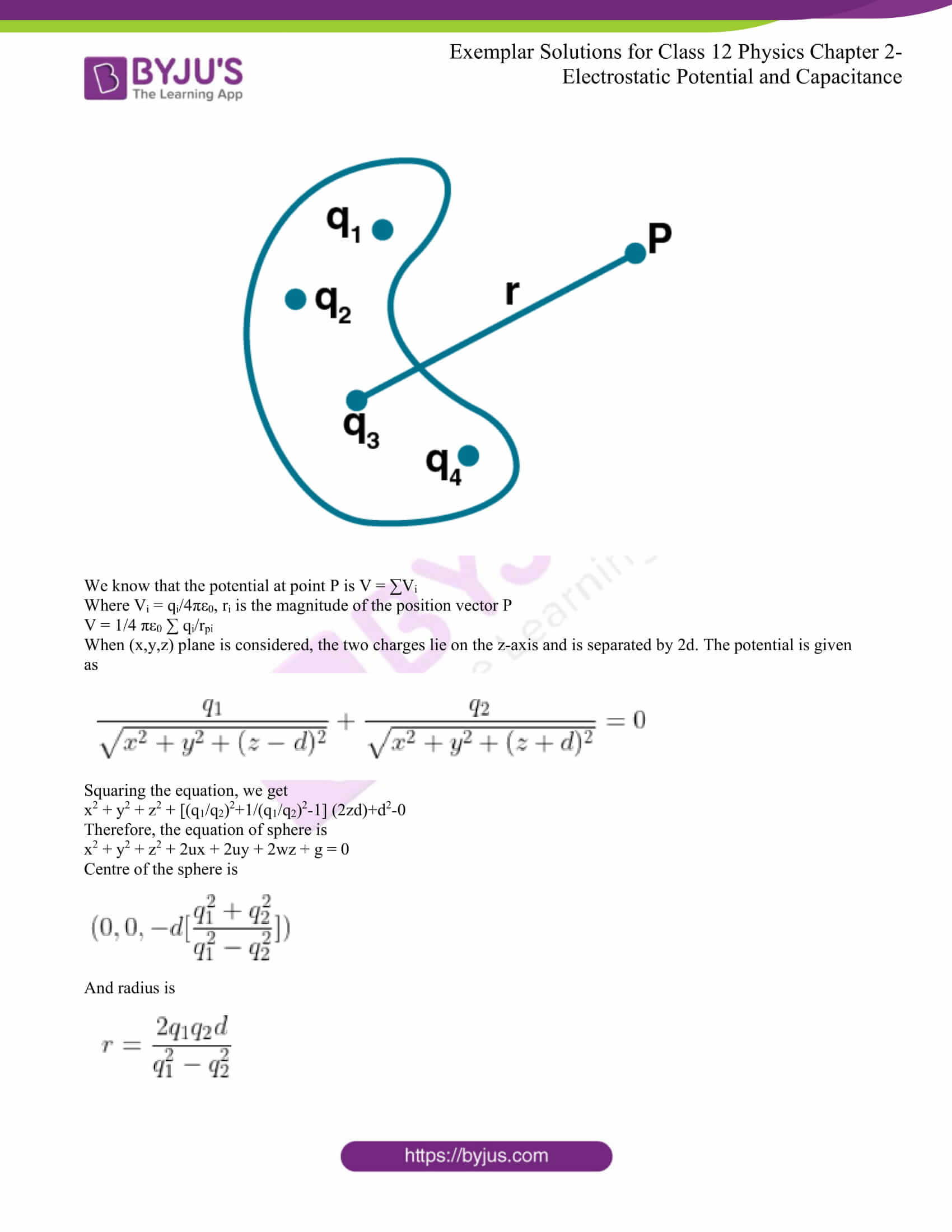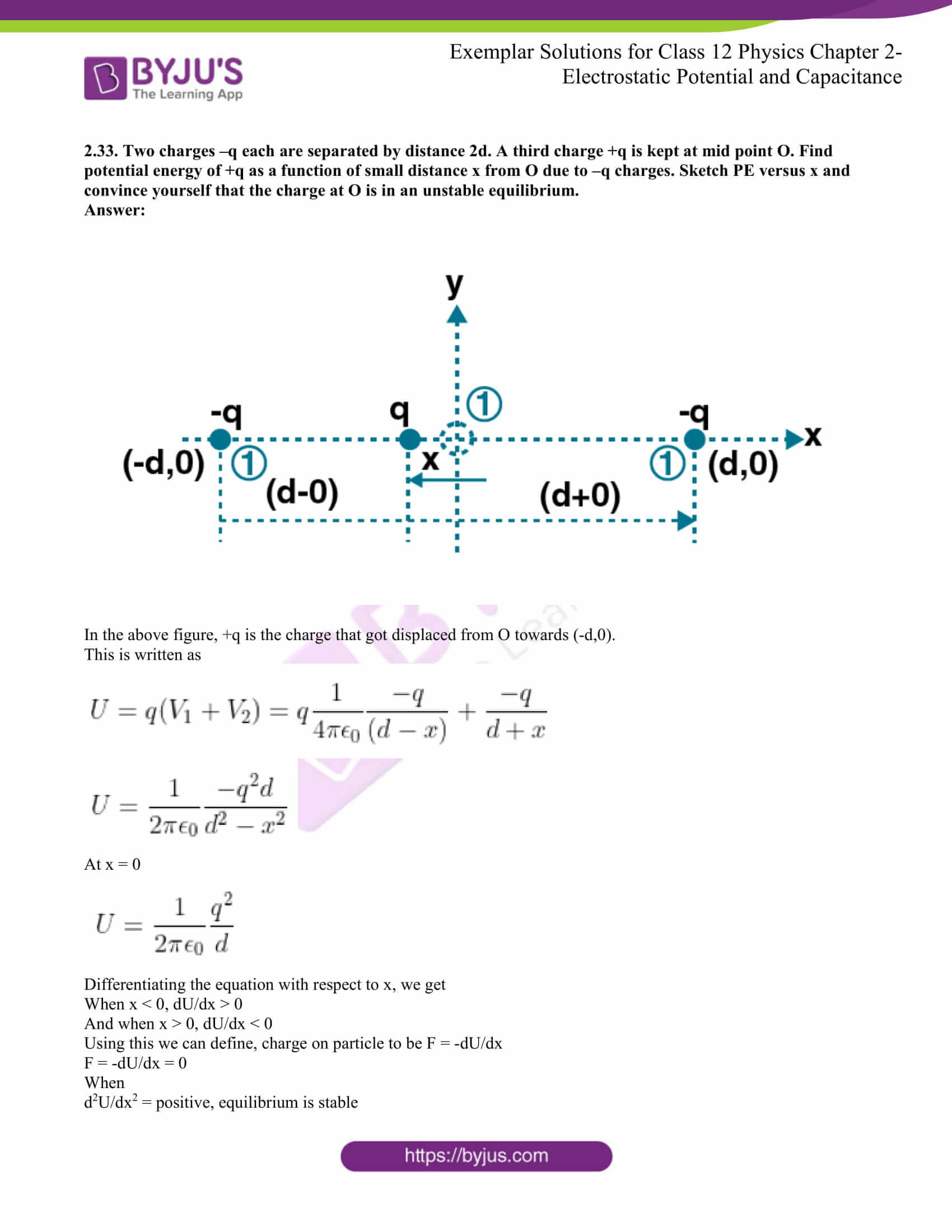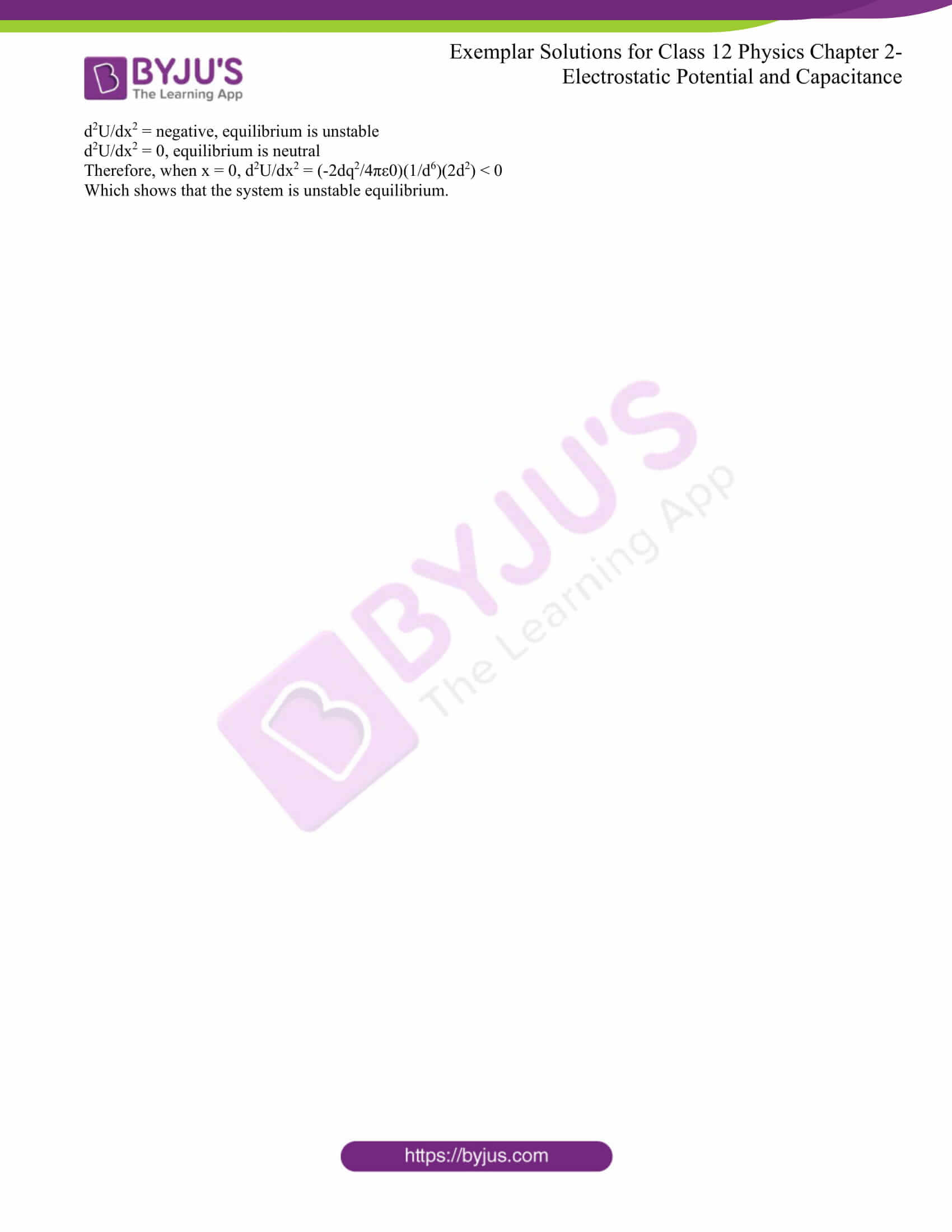### Multiple Choice Questions I

2.1. A capacitor of 4μF is connected, as shown in the circuit. The internal resistance of the battery is 0.5Ω. The amount of charge on the capacitor plates will be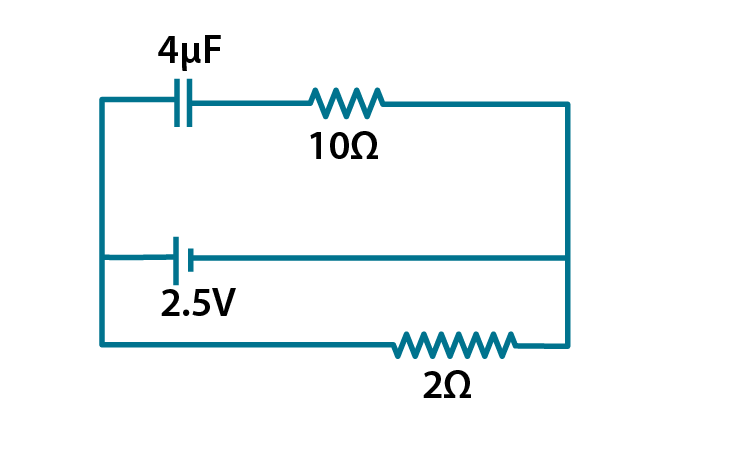a) 0

b) 4μC

c) 16μC

d) 8μC

The correct answer is d) 8μC

2.2. A positively charged particle is released from rest in a uniform electric field. The electric potential energy of the charge

a) remains a constant because the electric field is uniform

b) increases because the charge moves along the electric field

c) decreases because the charge moves along the electric field

d) decreases because the charge moves opposite to the electric field

The correct answer is c) decreases because the charge moves along the electric field

2.3. Figure shows some equipotential lines distributed in space. A charged object is moved from point A to point B.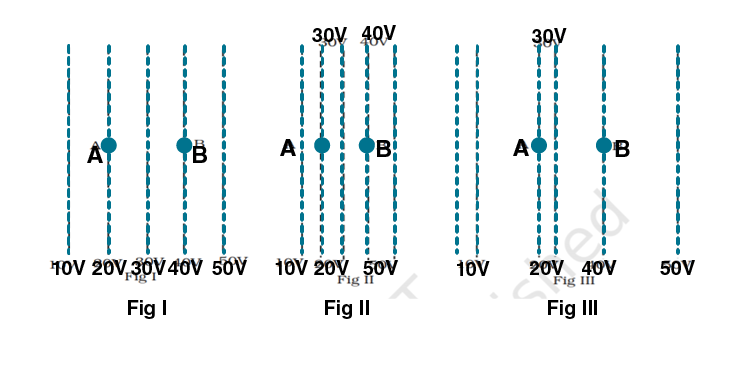a) the work done in fig (i) is the greatest

b) the work done in fig (ii) is the least

c) the work done is the same in fig (i), fig (ii), and fig (iii)

d) the work done in fig (iii) is greater than fig (ii) but equal to that in fig (i)

The correct answer is c) the work done is the same in fig (i), fig (ii), and fig (iii)

2.4. The electrostatic potential on the surface of a charged conducting sphere is 100V. Two statements are made in this regard:

S1: At any point inside the sphere, the electric intensity is zero

S2: At any point inside the sphere, the electrostatic potential is 100V

Which of the following is a correct statement?

a) S1 is true but S2 is false

b) Both S1 and S2 are false

c) S1 is true, S2 is also true, and S1 is the cause of S2

d) S1 is true, and S2 is also true, but the statements are independent

The correct answer is c) S1 is true, S2 is also true, and S1 is the cause of S2

2.5. Equipotential at a great distance from a collection of charges whose total sum is not zero are approximately

a) spheres

b) planes

c) paraboloids

d) ellipsoids

The correct answer is a) spheres

2.6. A parallel plate capacitor is made of two dielectric blocks in series. One of the blocks has thickness d1 and dielectric constant k1, and the other has thickness d2 and dielectric constant k2 as shown in the figure. This arrangement can be thought of as a dielectric slab of thickness d = d1 + d2 and effective dielectric constant k. The k is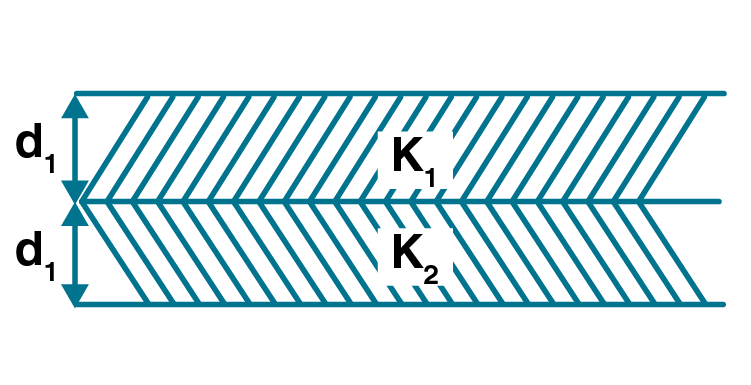a) k1d1 + k2d2/d1+d2

b) k1d1 + k2d2/k1 + k2

c) k1k2 (d1 + d2)/(k1d1 + k2d2)

d) 2k1k2/k1 + k2

The correct answer is c) k1k2 (d1 + d2)/(k1d1 + k2d2)

### Multiple Choice Questions II

2.7. Consider a uniform electric field in the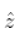direction. The potential is a constant

a) in all space

b) for any x for a given z

c) for any y for a given z

d) on the x-y plane for a given z

b) for any x for a given z

c) for any y for a given z

d) on the x-y plane for a given z

2.8. Equipotential surfaces

a) are closer in regions of large electric fields compared to regions of lower electric fields

b) will be more crowded near the sharp edges of a conductor

c) will be more crowded near regions of large charge densities

d) will always be equally spaced

a) are closer in regions of large electric fields compared to regions of lower electric fields

b) will be more crowded near the sharp edges of a conductor

c) will be more crowded near regions of large charge densities

2.9. The work done to move a charge along an equipotential from A to B

a) cannot be defined as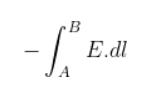b) must be defined asc) is zero

d) can have a non-zero value

b) must be defined asc) is zero

2.10. In a region of constant potential

a) the electric field is uniform

b) the electric field is zero

c) there can be no charge inside the region

d) the electric field shall necessarily change if a charge is placed outside the region

b) the electric field is zero

c) there can be no charge inside the region

2.11. In the circuit shown in the figure, initially, key K1 is closed, and K2 is open. Then K1 is opened, and K2 is closed. Then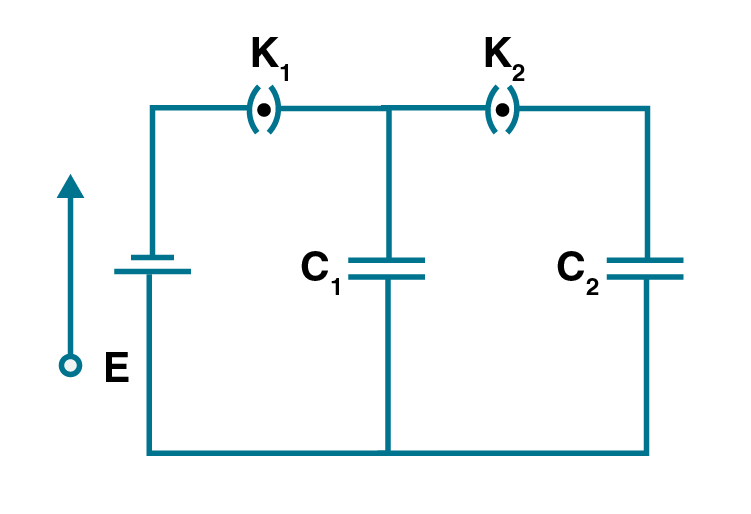a) charge on C1 gets redistributed such that V1 = V2

b) charge on C1 gets redistributed such that Q1’ = Q2

c) charge on C1 gets redistributed such that C1V1 + C2V2 = C1E

d) charge on C1 gets redistributed such that Q1’ + Q2’ = Q

a) charge on C1 gets redistributed such that V1 = V2

d) charge on C1 gets redistributed such that Q1’ + Q2’ = Q

2.12. If a conductor has a potential V ≠ 0 and there are no charges anywhere else outside, then

a) there must be charges on the surface or inside itself

b) there cannot be any charge in the body of the conductor

c) there must be charges only on the surface

d) there must be charges inside the surface

a) there must be charges on the surface or inside itself

b) there cannot be any charge in the body of the conductor

2.13. A parallel plate capacitor is connected to a battery, as shown in the figure. Consider two situations:

A: Key K is kept closed, and plates of capacitors are moved apart using the insulating handle

B: Key K is opened, and plates of capacitors are moved apart using the insulating handle

Choose the correct options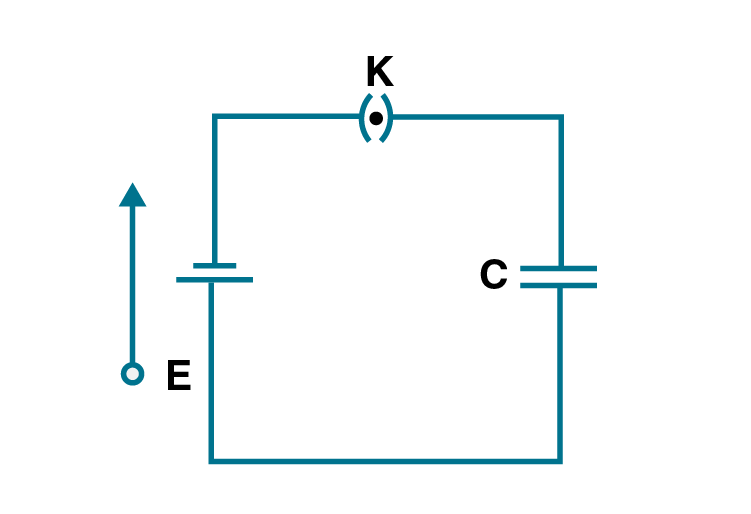a) In A: Q remains the same but C changes

b) In B: V remains the same, but C changes

c) In A: V remains the same, and hence Q changes

d) In B: Q remains the same, and hence V changes

c) In A: V remains the same, and hence Q changes

d) In B: Q remains the same, and hence V changes

2.14. Consider two conducting spheres of radii R1 and R2 with R1 > R2. If the two are at the same potential, the larger sphere has more charge than the smaller sphere. State whether the charge density of the smaller sphere is more or less than that of the larger one.

There are two spheres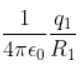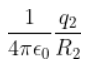σ1R1 = σ2R2

σ1/ σ2 = R2/R1

As R2 > R1, that means σ1 > σ2

Therefore, the charge density of the smaller sphere is more than that of the larger sphere.

2.15. Do free electrons travel to a region of higher potential or lower potential?

The force on the charged particle in the electric field is F = qE

The direction of the electric field and the direction of electrostatic force experienced by the free electrons are in opposite directions

The direction of the electric field is higher than the potential, and therefore, the electrons travel from a lower potential region to a higher potential.

2.16. Can there be a potential difference between two adjacent conductors carrying the same charge?

Yes, there can be a potential difference between two adjacent conductors carrying the same charge.

2.17. Can the potential function have a maximum or minimum in free space?

No, the potential function cannot be maximum or minimum in free space as the absence of atmosphere around the conductor prevents the electric discharge.

2.18. A test charge q is made to move in the electric field of a point charge Q along two different closed paths. The first path has sections along and perpendicular to lines of the electric field, and the second path is a rectangular loop of the same area as the first loop. How does the work done compare in the two cases?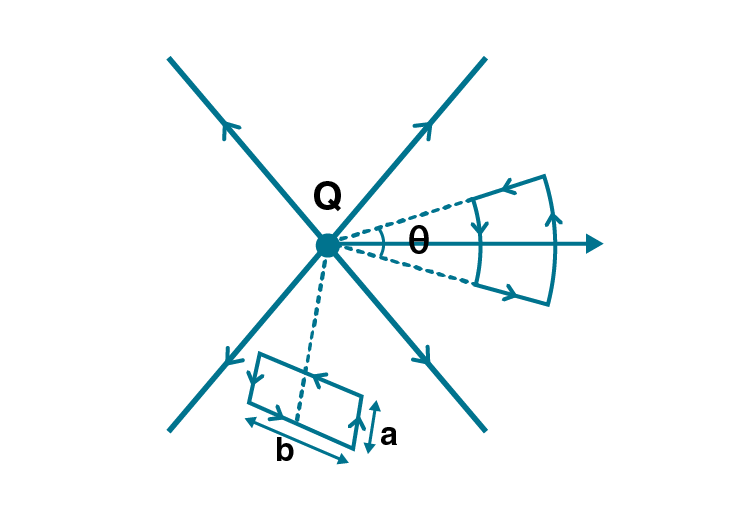Work done is zero in both cases. The work done by the electric force on the charge is in the closed loop, which is equal to zero.

2.19. Prove that a closed equipotential surface with no charge within itself must enclose an equipotential volume.

In a closed equipotential surface, the potential changes from position to position.

The potential inside the surface is different from the potential gradient caused in the surface that is dV/dr

This also means that the electric field is not equal to zero, and it is given as E = -dV/dr

Therefore, it could be said that the field lines are either pointing inwards or outwards the surface.

So, it can be said that the field lines originate from the charges inside, which contradicts the original assumption. Therefore, the volume inside the surface must be equipotential.

2.20. A capacitor has some dielectric between its plates, and the capacitor is connected to a DC source. The battery is now disconnected, and then the dielectric is removed. State whether the capacitance, energy stored in it, electric field, the charge stored, and voltage will increase, decrease, or remain constant.

 Quantity Battery is removed Battery remains connected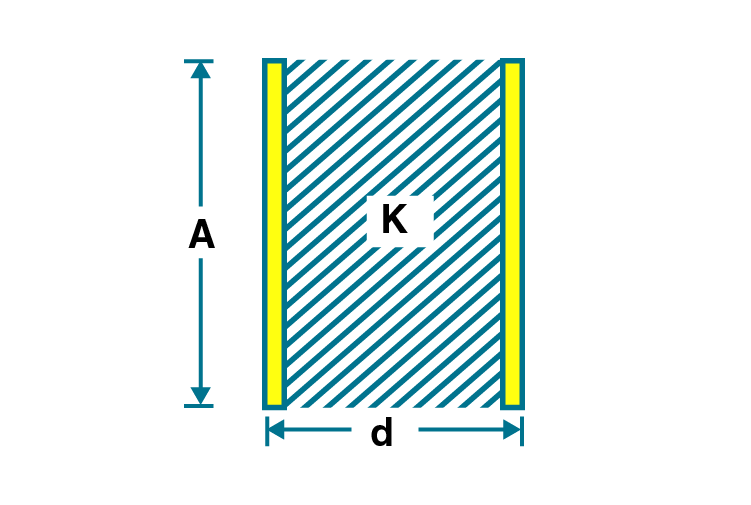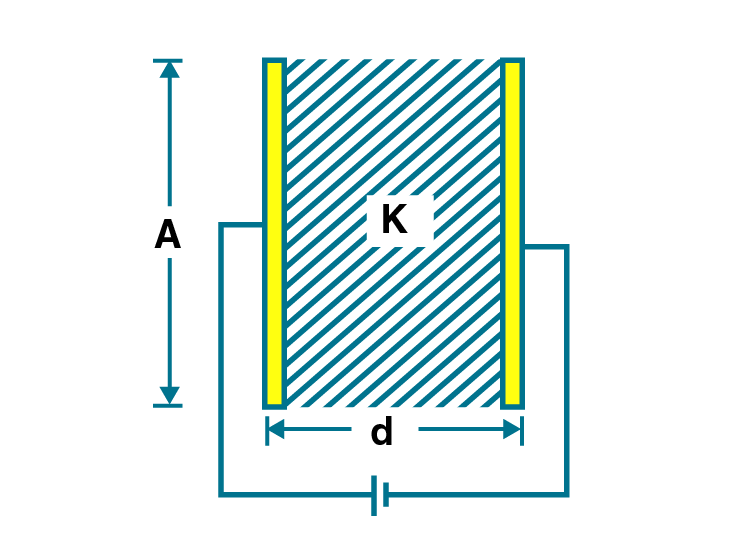Capacity C’ = KC C’ = KC Charge Q’ = Q Q’ = KQ Potential V’ = V/K V’ V Intensity E’ = E/K E’ = E Energy U’ = U/K U’ = UK

2.21. Prove that if an insulated, uncharged conductor is placed near a charged conductor and no other conductors are present, the uncharged body must be intermediate in potential between that of the charged body and that of infinity.

The electric potential decreasing along the direction of the electric field is given as E = dV/dr

The electric potential decreases when the path from the charged conductor is taken to the uncharged conductor along the direction of the electric field.

This continues when another uncharged conductor is considered to the infinity lowering the potential even further.

This shows that the uncharged body is at intermediate potential, and the charged body is at infinity potential.

2.22. Calculate the potential energy of a point charge –q placed along the axis due to charge +Q uniformly distributed along a ring of radius R. Sketch PE as a function of axial distance z from the centre of the ring. Looking at the graph, can you see what would happen if –q is displaced slightly from the centre of the ring?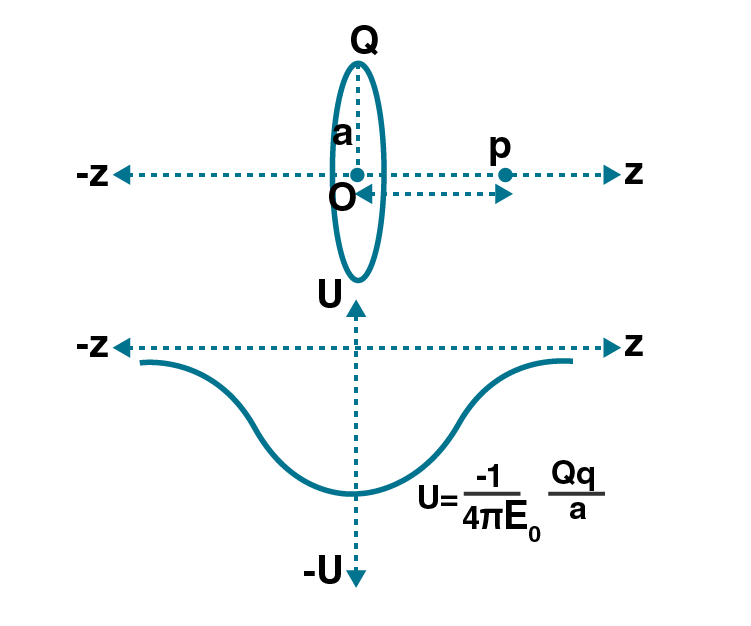U is the potential energy of a point charge q which is placed at potential V, U = qV

A negatively charged particle is placed at the axis of the ring with charge Q

Let a be the radius of the ring

The electric potential at the axial distance is given as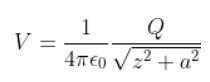The potential energy, U is given as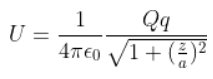When z = infinity, U = 0.

When z = 0, U is given as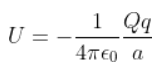2.23. Calculate potential on the axis of a ring due to charge Q uniformly distributed along the ring of radius R.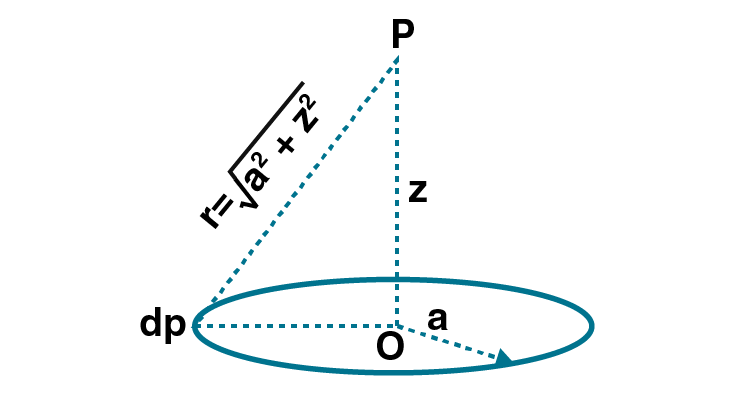Point P is considered to be at a distance z from the centre of the ring. The charge dq is at a distance z from point P.

V is written as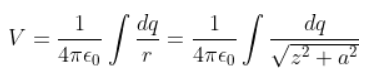Therefore, the net potential is given as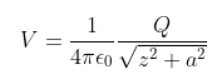2.24. Find the equation of the equipotential for an infinite cylinder of radius r0, carrying charge of linear density λ.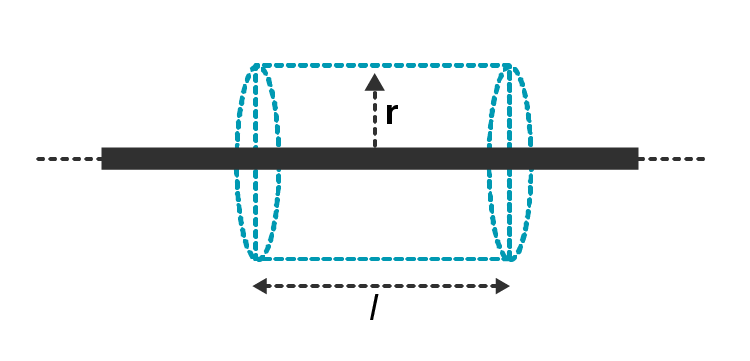The equation of the equipotential for an infinite cylinder of radius r0 with linear charge density λ is given as:

r = r0e 2πε0/ λ [V(r) – V(r0)]

2.25. Two-point charges of magnitude +q and –q are placed at (-d/2, 0, 0) and (d/2, 0, 0), respectively. Find the equation of the equipotential surface where the potential is zero.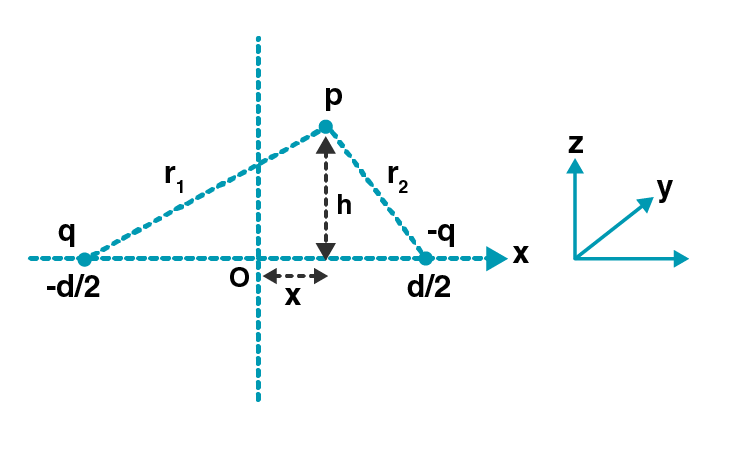The potential at point P due to charges is given as

Vp = 1/4πε0 q/r1 + 1/4 πε0 (-q)/r2

The net electric potential at this point is zero,

Therefore, r1 = r2

We know that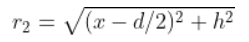To solve the two equations, we require the equation in-plane x = 0, which is a y-z plane.

2.26. A parallel plate capacitor is filled by a dielectric whose relative permittivity varies with the applied voltage (U) as ε = αU where α = 2V-1. A similar capacitor with no dielectric is charged to U0 = 78 V. It is then connected to the uncharged capacitor with the dielectric. Find the final voltage on the capacitors.

Since the capacitors are connected in parallel, the potential difference across the capacitors is the same. The final voltage is assumed to be U. C is the capacitance of the capacitor without dielectric, then the charge is given as Q1 = CU

The initial charge is given as

Q0 = CU0

The conversion of charges is

Q0 = Q1 + Q2

CU0 = CU + αCU2

αU2 + U – U0 = 0

Solving the equation, we get U = 6V

2.27. A capacitor is made of two circular plates of radius R each, separated by a distance d < < R. The capacitor is connected to a constant voltage. A thin conducting disc of radius r < < R and thickness t < < r is placed at the centre of the bottom plate. Find the minimum voltage required to lift the disc if the mass of the disc is m.

When the conducting disc is placed at the centre of the bottom plate, the potential of the disc is equal to the potential of the plate. The electric field on the disc is given as

E = V/d

The charge q’ is transferred to the disc, which is given as

q’ = -ε0 V/d πr2

The force acting on the disc is

F = ε0 V2/d2 πr2

Therefore, V = square root of mdg/ π ε0r2

2.28. a) In a quark model of elementary particles, a neutron is made of one up quark and two down quarks. Assume that they have a triangle configuration with a side length of the order of 10-15 m. Calculate the electrostatic potential energy of the neutron and compare it with its mass 939 MeV.

b) Repeat the above exercise for a proton which is made of two up and one down quarks.

There are three charges in the system. The potential energy of the system is equal to the sum of the PE of each pair.

U = 1/4 π ε0 {qdqd/r – quqd/r – quqd/r}

Substituting the values we get,

U = 5.11 10-4

2.29. Two metal spheres, one of radius R and the other of radius 2R, both have the same surface charge density σ. They are brought in contact and separated. What will be the new surface charge densities on them?

Following are the charges on the metal sphere before contact

Q1 = σ.4πR2

Q2 = σ.4π(2R)2 = 4Q1

Q1’ and Q2’ are the charges on the metal sphere after contact

Q1’ + Q2’ = Q1 + Q2 = 5Q1

When the metal spheres are in contact, the following is the potentials acquired by them

Q1’ = Q2’/2

Solving the equations we

σ1 = 5 σ/3

σ2 = 5 σ/6

2.30. In the circuit shown in the figure, initially, K1 is closed, and K2 is open. What are the charges on each capacitor? Then K1 was opened, and K2 was closed. What will be the charge on each capacitor now?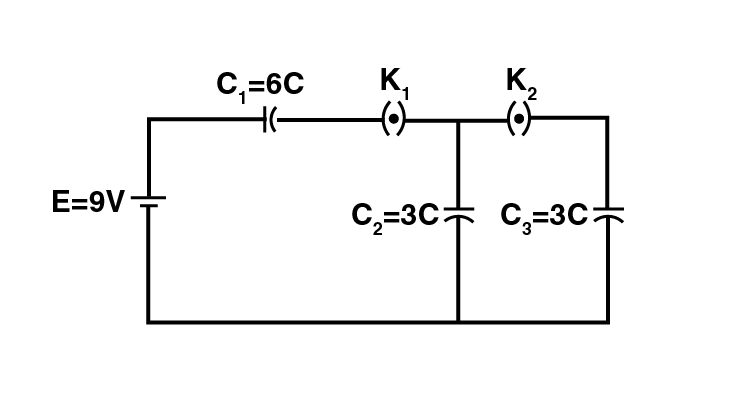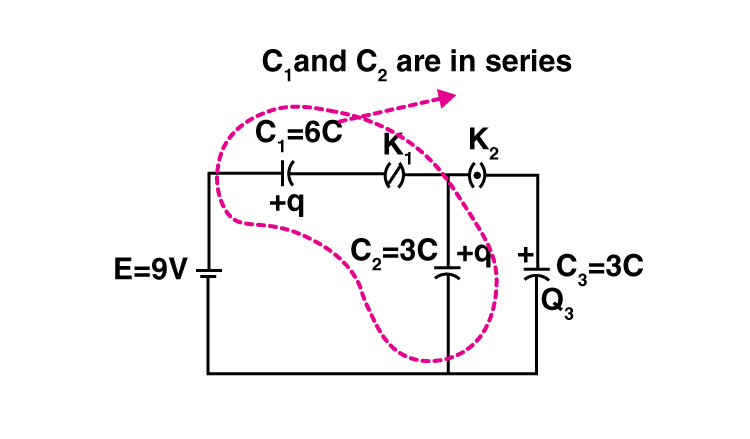When K1 is closed, and K2 is open, the capacitors C1 and C2 are connected in series with the battery

Therefore, the charge in capacitors C1 and C2 are

Q1 = Q2 = q = (C1C2/C1+C2)E = 18μC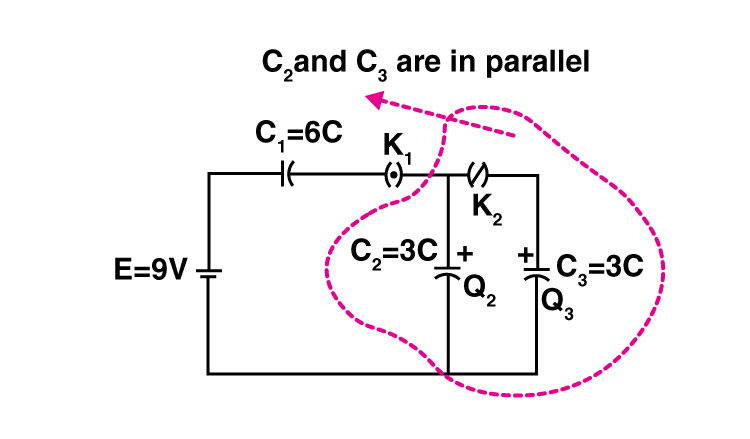When the capacitors C2 and C3 are placed in parallel,

C2V’ + C3V’ = Q2

V’ = Q2/C2 + C3 = 3V

Therefore,

Q2’ = 3CV’ = 9 μC

Q3’ = 3CV’ = μC

Q1’ = 18 μC

2.31. Calculate potential on the axis of a disc of radius R due to a charge Q uniformly distributed on its surface.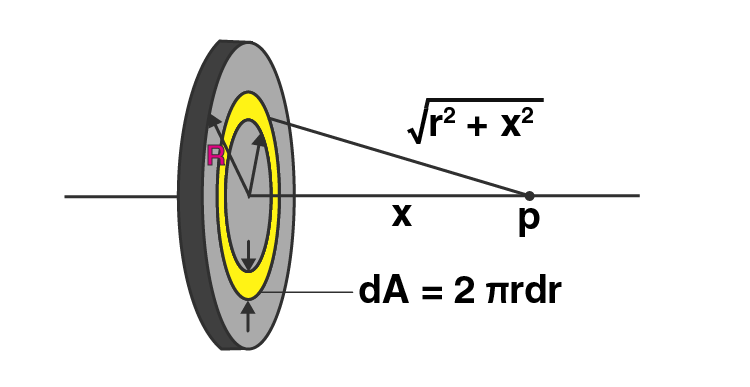In the above figure, we can see that the disc is divided into several charged rings. Let P be the point on the axis of the disc at a distance x from the centre of the disc.

The radius of the ring is r and the width is dr. dq is the charge on the ring, which is given as

dq = σdA = σ2πrdr

The potential is given as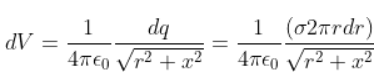The total potential at P is given as

Q/2πε0R2 (√R2 + x2 –x)

2.32. Two charges, q1 and q2, are placed at (0, 0, d) and (0, 0, -d), respectively. Find the locus of points where the potential is zero.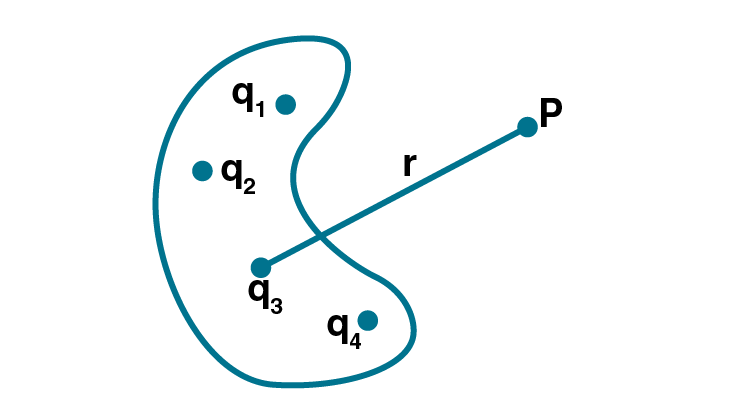We know that the potential at point P is V = ∑Vi

Where Vi = qi/4πε0, ri is the magnitude of the position vector P

V = 1/4 πε0 ∑ qi/rpi

When the (x,y,z) plane is considered, the two charges lie on the z-axis and are separated by 2d. The potential is given as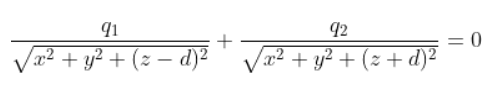Squaring the equation, we get

x2 + y2 + z2 + [(q1/q2)2+1/(q1/q2)2-1] (2zd)+d2-0

Therefore, the equation of the sphere is

x2 + y2 + z2 + 2ux + 2uy + 2wz + g = 0

The centre of the sphere is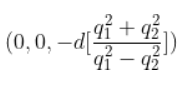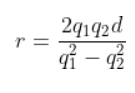2.33. Two charges –q each are separated by distance 2d. A third charge +q is kept at midpoint O. Find potential energy of +q as a function of small distance x from O due to –q charges. Sketch PE versus x and convince yourself that the charge at O is in an unstable equilibrium.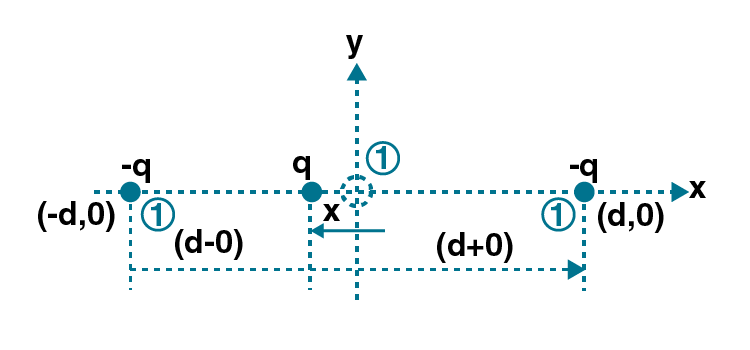In the above figure, +q is the charge that got displaced from O towards (-d,0).

This is written as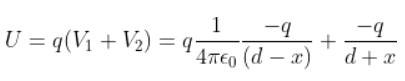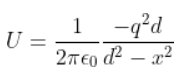At x = 0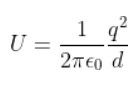Differentiating the equation with respect to x, we get

When x < 0, dU/dx > 0

And when x > 0, dU/dx < 0

Using this, we can define the charge on the particle to be F = -dU/dx

F = -dU/dx = 0

When

d2U/dx2 = positive, equilibrium is stable

d2U/dx2 = negative, equilibrium is unstable

d2U/dx2 = 0, equilibrium is neutral

Therefore, when x = 0, d2U/dx2 = (-2dq2/4πε0)(1/d6)(2d2) < 0

Which shows that the system is in unstable equilibrium.

Electric charges which are slow or stationary is called Electrostatic Charge, and the amount of work needed to move from one initial point to another is termed Electrostatic Potential. It can be measured in volts and is a scalar quantity. Capacitance, on the other hand, can be defined as the ability of a capacitor to store energy. They are measured in farads.

### Important Concepts Covered in NCERT Exemplar Electrostatic Potential and Capacitance

 Sl.No. Concept Name 1 Electrostatic Potential – derivation of the electric formula 2 Potential Due to a Point Charge – relation between electric charge and potential 3 Potential Due to an Electric Dipole – derivation of potential access formula 4 Potential Due to a System of Charges – derivation of the potential energy of a system of two charges 5 Equipotential Surfaces – derivation of potential due to charge 6 The Relation between Field and Potential: Potential Energy of a System of Charges Potential Energy in an External Field Potential Energy of a Single Charge 7 Potential Energy of a System of Two Charges in an External Field 8 Potential Energy of a Dipole in an External Field 9 Electrostatics of Conductors in Different Conditions, Electro-shielding 10 Dielectrics and Polarisation – meaning dielectric constant derivation 11 Capacitors and Capacitance – meaning, capacitance derivation and problems 12 The Parallel-Plate Capacitor – meaning and derivation 13 Effect of Dielectric on Capacitance – derivations and numerical problems 14 Combination of Capacitors – capacitors in series, capacitors in parallel 15 Energy Stored in a Capacitor – Energy density of electric field, derivation 16 Van De Graaff Generator – introduction, applications

Why Opt for BYJU’S?

BYJU’S top-notch faculty, having high experience and talent in the respective field, will guide students in learning the concepts and topics thoroughly and memorising them for a very long time. BYJU’S interactive teaching methods include videos, animations, info-graphics, study materials, notes, tips and tricks, which will help students in scoring good marks in the Class 12 board examination.